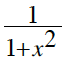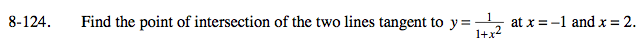### Home > CALC > Chapter 8 > Lesson 8.3.3 > Problem8-124

8-124.

Find the point of intersection of the two lines tangent to y =at x = −1 and x = 2. Homework Help ✎Tangent at x = − 1:

Find tangent at x = 2.

Solve a system of equations to find the point-of-intersection between the two tangents.